This book is archived and will be removed July 6, 2022. Please use the updated version.

Standard Costs and Variances

# 48 Compute and Evaluate Overhead Variances

Recall that the standard cost of a product includes not only materials and labor but also variable and fixed overhead. It is likely that the amounts determined for standard overhead costs will differ from what actually occurs. This will lead to overhead variances.

### Determination and Evaluation of Overhead Variance

In a standard cost system, overhead is applied to the goods based on a standard overhead rate. This is similar to the predetermined overhead rate used previously. The standard overhead rate is calculated by dividing budgeted overhead at a given level of production (known as normal capacity) by the level of activity required for that particular level of production.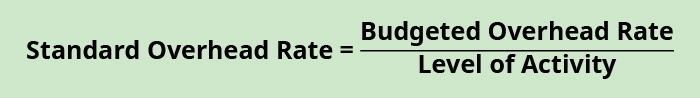Usually, the level of activity is either direct labor hours or direct labor cost, but it could be machine hours or units of production.

#### Creation of Flexible Overhead Budget

To determine the overhead standard cost, companies prepare a flexible budget that gives estimated revenues and costs at varying levels of production. The standard overhead cost is usually expressed as the sum of its component parts, fixed and variable costs per unit. Note that at different levels of production, total fixed costs are the same, so the standard fixed cost per unit will change for each production level. However, the variable standard cost per unit is the same per unit for each level of production, but the total variable costs will change.

We continue to use Connie’s Candy Company to illustrate. Suppose Connie’s Candy budgets capacity of production at 100% and determines expected overhead at this capacity. Connie’s Candy also wants to understand what overhead cost outcomes will be at 90% capacity and 110% capacity. The following information is the flexible budget Connie’s Candy prepared to show expected overhead at each capacity level.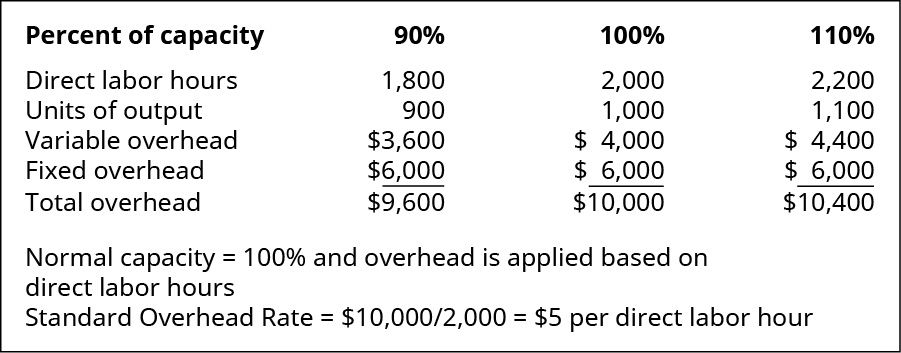Using the flexible budget, we can determine the standard variable cost per unit at each level of production by taking the total expected variable overhead divided by the level of activity, which can still be direct labor hours or machine hours.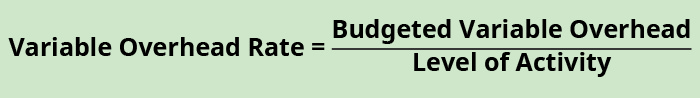Looking at Connie’s Candies, the following table shows the variable overhead rate at each of the production capacity levels.

 Production Capacity Variable/Unit 90% ?3,600/1,800 = ?2 100% ?4,000/2,000 = ?2 110% ?4,400/2,200 = ?2

Sometimes these flexible budget figures and overhead rates differ from the actual results, which produces a variance.

#### Determination of Variable Overhead Variances

There are two components to variable overhead rates: the overhead application rate and the activity level against which that rate was applied. If we compare the actual variable overhead to the standard variable overhead, by analyzing the difference between actual overhead costs and the standard overhead for current production, it is difficult to determine if the variance is due to application rate differences or activity level differences. Thus, there are two variable overhead variances that will better provide these answers: the variable overhead rate variance and the variable overhead efficiency variance.

#### Determination of Variable Overhead Rate Variance

The variable overhead rate variance, also known as the spending variance, is the difference between the actual variable manufacturing overhead and the variable overhead that was expected given the number of hours worked. The variable overhead rate variance is calculated using this formula: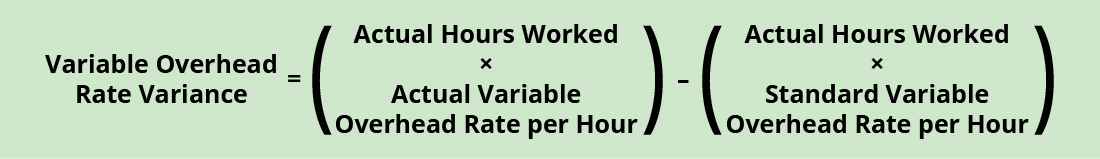Factoring out actual hours worked, we can rewrite the formula asIf the outcome is favorable (a negative outcome occurs in the calculation), this means the company spent less than what it had anticipated for variable overhead. If the outcome is unfavorable (a positive outcome occurs in the calculation), this means the company spent more than what it had anticipated for variable overhead.

Connie’s Candy Company wants to determine if its variable overhead spending was more or less than anticipated. Connie’s Candy had this data available in the flexible budget: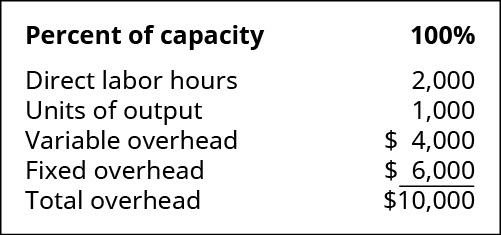Connie’s Candy also had this actual output information: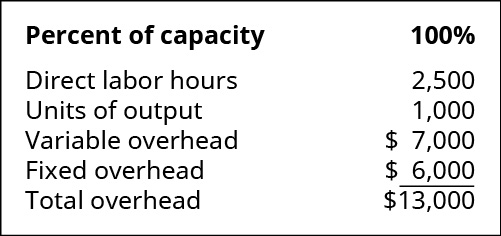To determine the variable overhead rate variance, the standard variable overhead rate per hour and the actual variable overhead rate per hour must be determined. The standard variable overhead rate per hour is ?2.00 (?4,000/2,000 hours), taken from the flexible budget at 100% capacity. The actual variable overhead rate is ?2.80 (?7,000/2,500), taken from the actual results at 100% capacity. Therefore,

$$\text{Variable Overhead Rate Variance}=\left(?2.80–?2.00\right)\phantom{\rule{0.2em}{0ex}}×\phantom{\rule{0.2em}{0ex}}2,500=?2,000\phantom{\rule{0.2em}{0ex}}\left(\text{Unfavorable}\right)$$

This produces an unfavorable outcome. This could be for many reasons, and the production supervisor would need to determine where the variable cost difference is occurring to make production changes.

Let us look at another example producing a favorable outcome. Connie’s Candy had this data available in the flexible budget: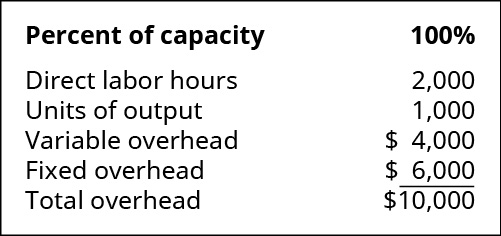Connie’s Candy also had this actual output information: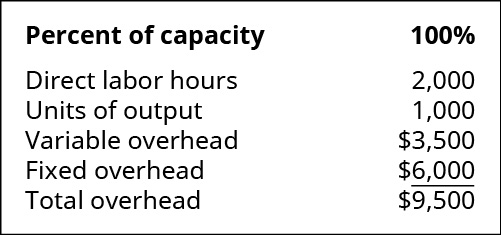To determine the variable overhead rate variance, the standard variable overhead rate per hour and the actual variable overhead rate per hour must be determined. The standard variable overhead rate per hour is ?2.00 (?4,000/2,000 hours), taken from the flexible budget at 100% capacity. The actual variable overhead rate is ?1.75 (?3,500/2,000), taken from the actual results at 100% capacity. Therefore,

$$\text{Variable Overhead Rate Variance}=\left(?1.75–?2.00\right)\phantom{\rule{0.2em}{0ex}}×\phantom{\rule{0.2em}{0ex}}?2,000=–?500\phantom{\rule{0.2em}{0ex}}\text{or}\phantom{\rule{0.2em}{0ex}}?500\phantom{\rule{0.2em}{0ex}}\left(\text{Favorable}\right)$$

This produces a favorable outcome. This could be for many reasons, and the production supervisor would need to determine where the variable cost difference is occurring to better understand the variable overhead reduction.

Interpretation of the variable overhead rate variance is often difficult because the cost of one overhead item, such as indirect labor, could go up, but another overhead cost, such as indirect materials, could go down. Often, explanation of this variance will need clarification from the production supervisor. Another variable overhead variance to consider is the variable overhead efficiency variance.

#### Determination of Variable Overhead Efficiency Variance

The variable overhead efficiency variance, also known as the controllable variance, is driven by the difference between the actual hours worked and the standard hours expected for the units produced. This variance measures whether the allocation base was efficiently used. The variable overhead efficiency variance is calculated using this formula: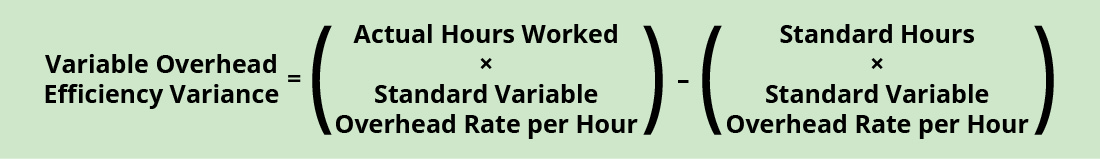Factoring out standard overhead rate, the formula can be written as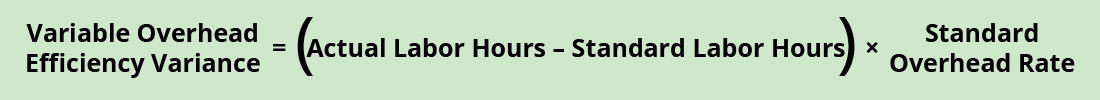If the outcome is favorable (a negative outcome occurs in the calculation), this means the company was more efficient than what it had anticipated for variable overhead. If the outcome is unfavorable (a positive outcome occurs in the calculation), this means the company was less efficient than what it had anticipated for variable overhead.

Connie’s Candy Company wants to determine if its variable overhead efficiency was more or less than anticipated. Connie’s Candy had the following data available in the flexible budget: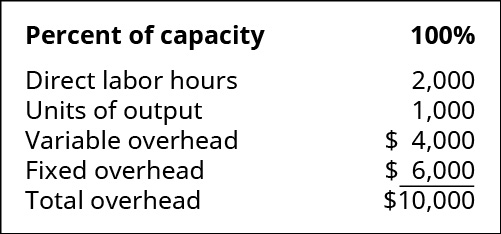Connie’s Candy also had the following actual output information: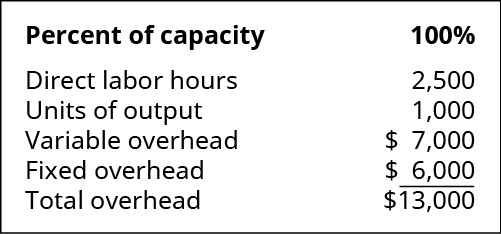To determine the variable overhead efficiency variance, the actual hours worked and the standard hours worked at the production capacity of 100% must be determined. Actual hours worked are 2,500, and standard hours are 2,000. The standard variable overhead rate per hour is ?2.00 (?4,000/2,000 hours), taken from the flexible budget at 100% capacity. Therefore,

$$\text{Variable Overhead Efficiency Variance}=\left(2,500–2,000\right)\phantom{\rule{0.2em}{0ex}}×\phantom{\rule{0.2em}{0ex}}?2.00=?1,000\phantom{\rule{0.2em}{0ex}}\left(\text{Unfavorable}\right)$$

This produces an unfavorable outcome. This could be for many reasons, and the production supervisor would need to determine where the variable cost difference is occurring to make production changes.

Let us look at another example producing a favorable outcome. Connie’s Candy had the following data available in the flexible budget: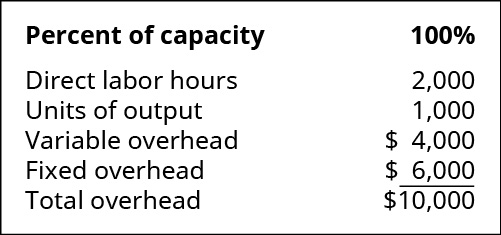Connie’s Candy also had the following actual output information: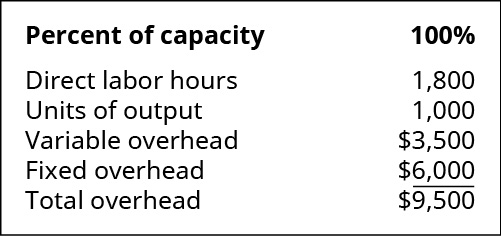To determine the variable overhead efficiency variance, the actual hours worked and the standard hours worked at the production capacity of 100% must be determined. Actual hours worked are 1,800, and standard hours are 2,000. The standard variable overhead rate per hour is ?2.00 (?4,000/2,000 hours), taken from the flexible budget at 100% capacity. Therefore,

$$\text{Variable Overhead Efficiency Variance}=\left(1,800–2,000\right)\phantom{\rule{0.2em}{0ex}}×\phantom{\rule{0.2em}{0ex}}?2.00=–?400\phantom{\rule{0.2em}{0ex}}\text{or}\phantom{\rule{0.2em}{0ex}}?400\phantom{\rule{0.2em}{0ex}}\left(\text{Favorable}\right)$$

This produces a favorable outcome. This could be for many reasons, and the production supervisor would need to determine where the variable cost difference is occurring to better understand the variable overhead efficiency reduction.

The total variable overhead cost variance is also found by combining the variable overhead rate variance and the variable overhead efficiency variance. By showing the total variable overhead cost variance as the sum of the two components, management can better analyze the two variances and enhance decision-making.

(Figure) shows the connection between the variable overhead rate variance and variable overhead efficiency variance to total variable overhead cost variance.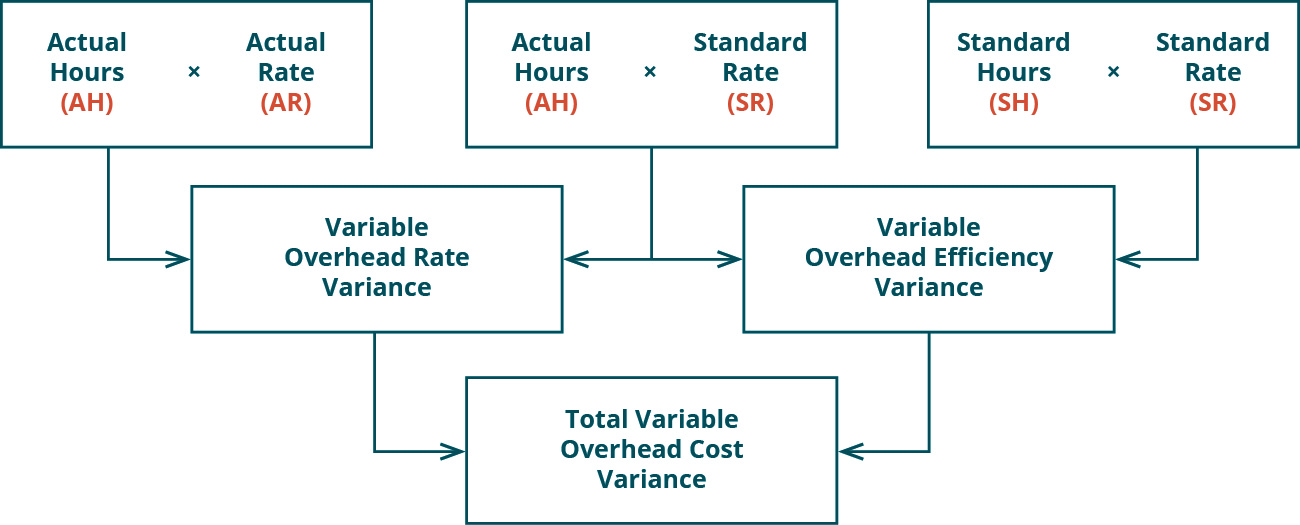For example, Connie’s Candy Company had the following data available in the flexible budget: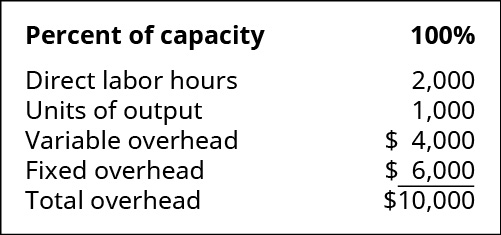Connie’s Candy also had the following actual output information: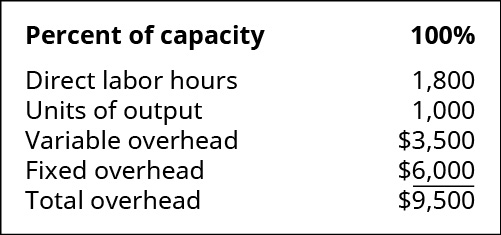The variable overhead rate variance is calculated as (1,800 × ?1.94) – (1,800 × ?2.00) = –?108, or ?108 (favorable). The variable overhead efficiency variance is calculated as (1,800 × ?2.00) – (2,000 × ?2.00) = –?400, or ?400 (favorable).

The total variable overhead cost variance is computed as:

$$\text{Total Variable Overhead Cost Variance}=\left(–?108\right)+\left(–?400\right)=–?508\phantom{\rule{0.2em}{0ex}}\text{or}\phantom{\rule{0.2em}{0ex}}?508\phantom{\rule{0.2em}{0ex}}\left(\text{Favorable}\right)$$

In this case, two elements are contributing to the favorable outcome. Connie’s Candy used fewer direct labor hours and less variable overhead to produce 1,000 candy boxes (units).

The same calculation is shown as follows in diagram format.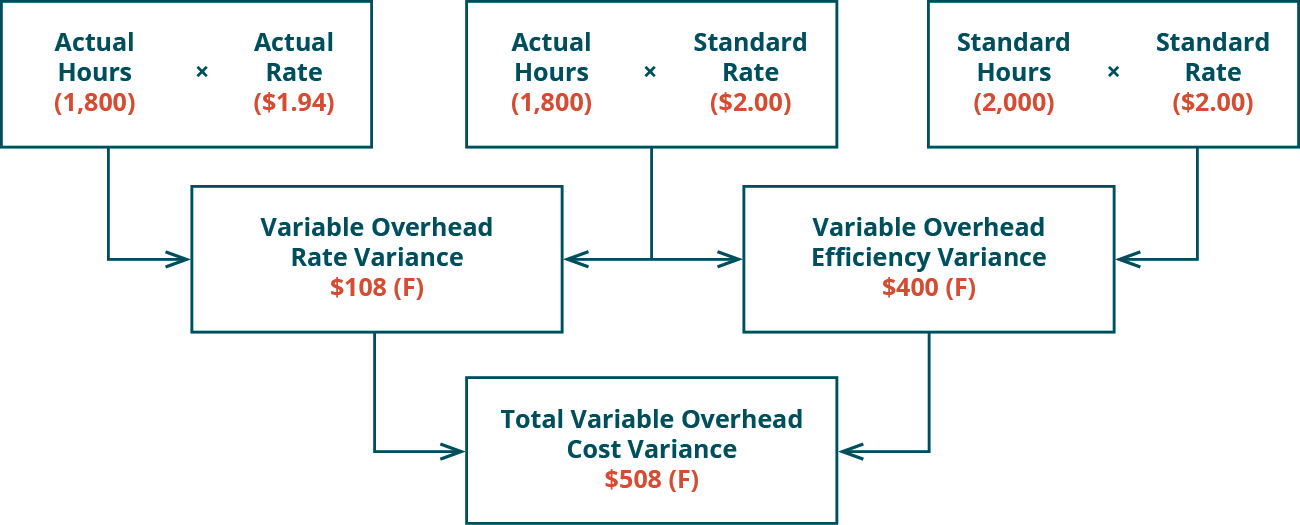As with the interpretations for the variable overhead rate and efficiency variances, the company would review the individual components contributing to the overall favorable outcome for the total variable overhead cost variance, before making any decisions about production in the future. Other variances companies consider are fixed factory overhead variances.

#### Fundamentals of Fixed Factory Overhead Variances

The fixed factory overhead variance represents the difference between the actual fixed overhead and the applied fixed overhead. There are two fixed overhead variances. One variance determines if too much or too little was spent on fixed overhead. The other variance computes whether or not actual production was above or below the expected production level.

Biglow Company makes a hair shampoo called Sweet and Fresh. They have the following flexible budget data: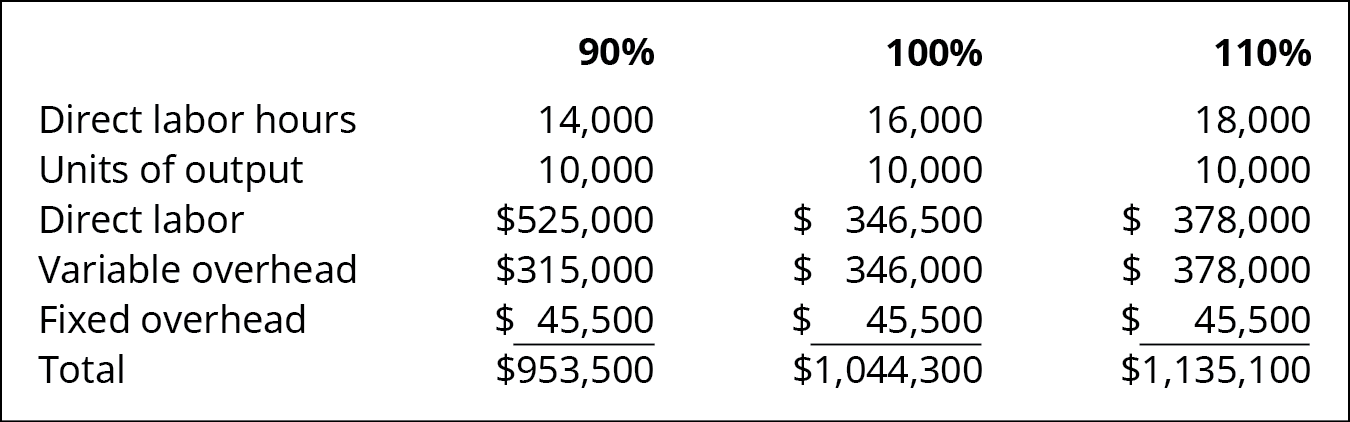What is the standard variable overhead rate at 90%, 100%, and 110% capacity levels?

Solution

90% = ?315,000/14,000 = ?22.50, 100% = ?346,000/16,000 = ?21.63 (rounded), 110% = ?378,000/18,000 = ?21.00.

The XYZ Firm is bidding on a contract for a new plane for the military. As the management team is going over the bid, they come to the conclusion it is too high on a per-plane basis, but they cannot find any costs they feel can be reduced. The information from the military states they will purchase between 50 and 100 planes, but will more likely purchase 50 planes rather than 100 planes. XYZ’s bid is based on 50 planes. The controller suggests that they base their bid on 100 planes. This would spread the fixed costs over more planes and reduce the bid price. The lower bid price will increase substantially the chances of XYZ winning the bid. Should XYZ Firm keep the bid at 50 planes or increase its bid to 100 planes? What are the pros and cons to keeping the bid at 50 or increasing to 100 planes?

### Key Concepts and Summary

• There are two sets of overhead variances: variable and fixed.
• The variable variances are caused by the overhead application rate and the activity level against which the rate was applied.
• The variable overhead rate variance is the difference between the actual variable manufacturing overhead and the variable overhead that was expected given the number of hours worked.
• The variable overhead efficiency variance is driven by the difference between the actual hours worked and the standard hours expected for the units produced.
• There are two fixed overhead variances. One is caused by spending too much or too little on fixed overhead. The other is caused by actual production being above or below the expected production level.

(Figure)A flexible budget ________.

1. predicts estimated revenues and costs at varying levels of production
2. gives actual figures for selling price
3. gives actual figures for variable and fixed overhead
4. is not used in overhead variance calculations

(Figure)The variable overhead rate variance is caused by the sum between which of the following?

1. actual and standard allocation base
2. actual and standard overhead rates
3. actual and budgeted units
4. actual units and actual overhead rates

B

(Figure)The variable overhead efficiency variance is caused by the difference between which of the following?

1. actual and budgeted units
2. actual and standard allocation base
3. actual and standard overhead rates
4. actual units and actual overhead rates

(Figure)The fixed factory overhead variance is caused by the difference between which of the following?

1. actual and standard allocation base
2. actual and budgeted units
4. actual and standard overhead rates

C

(Figure)What causes the variable overhead rate variance?

(Figure)What causes the variable overhead efficiency variance?

The difference between the actual and standard amounts of the allocation base cause variable overhead efficiency variance.

(Figure)What is the main difference between a flexible budget and a master budget?

(Figure)ThingOne Company has the following information available for the past year. They use machine hours to allocate overhead.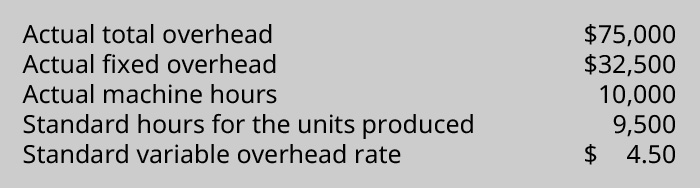What is the variable overhead efficiency variance?

(Figure)A manufacturer planned to use ?78 of variable overhead per unit produced, but in the most recent period, it actually used ?76 of variable overhead per unit produced. During this same period, the company planned to produce 500 units but actually produced 540 units. What is the variable overhead spending variance?

(Figure)A manufacturer planned to use ?45 of variable overhead per unit produced, but in the most recent period, it actually used ?47 of variable overhead per unit produced. During this same period, the company planned to produce 200 units but actually produced 220 units. What is the variable overhead spending variance?

(Figure)Fitzgerald Company manufactures sewing machines, and they produced 2,500 this past month. The standard variable manufacturing overhead (MOH) rate used by the company is ?6.75 per machine hour. Each sewing machine requires 13.5 machine hours. Actual machine hours used last month were 33,500, and the actual variable MOH rate last month was ?7.00.

Calculate the variable overhead rate variance and the variable overhead efficiency variance.

(Figure)Prepare a flexible budget for overhead based on the following data: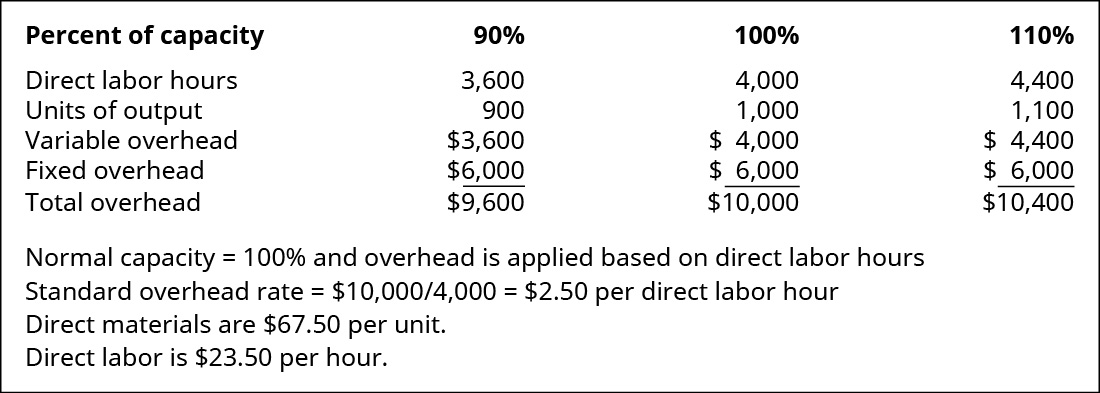(Figure)Reddy Corporation has collected the following data for the month of June: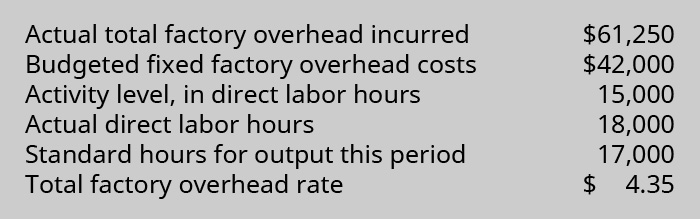What is the variable overhead efficiency variance?

(Figure)ABC Inc. spent a total of ?48,000 on factory overhead. Of this, ?28,000 was fixed overhead. ABC Inc. had budgeted ?27,000 for fixed overhead. Actual machine hours were 5,000. Standard hours for units made were 4,800. The standard variable overhead rate was ?4.10. What is the variable overhead rate variance?

(Figure)Why would managers use a flexible budget? What information does it provide that a regular budget does not?

(Figure)Fill in the blanks in the following flexible budget: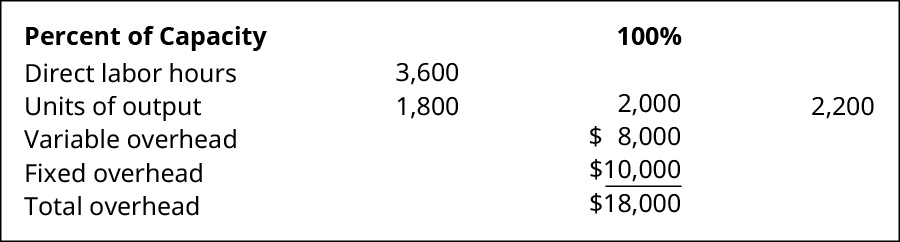(Figure)Before automation became more prevalent, overhead was often calculated and allocated as a function of direct labor costs or direct labor hours. Why was this the case, and has this pattern changed?

### Glossary

flexible budget
measurement and prediction of estimated revenues and costs at varying levels of production